## Zooming in on the HiggsMarch 24, 2009

Posted by dorigo in news, physics, science.
Tags: , , , , , , , , ,Yesterday Sven Heinemeyer kindly provided me with an updated version of a plot which best describes the experimental constraints on the Higgs boson mass, coming from electroweak observables measured at LEP and SLD, and from the most recent measurements of W boson and top quark masses. It is shown on the right (click to get the full-sized version).

The graph is a quite busy one, but I will try below to explain everything one bit at a time, hoping I keep things simple enough that a non-physicist can understand it.

The axes show suitable ranges of values of the top quark mass (varying on the horizontal axis) and of the W boson masses (on the vertical axis). The value of these quantities is functionally dependent (because of quantum effects connected to the propagation of the particles and their interaction with the Higgs field) on the Higgs boson mass.

The dependence, however, is really “soft”: if you were to double the Higgs mass by a factor of two from its true value, the effect on top and W masses would be only of the order of 1% or less. Because of that, only recently have the determinations of top quark and W boson masses started to provide meaningful inputs for a guess of the mass of the Higgs.

Top mass and W mass measurements are plotted in the graphs in the form of ellipses encompassing the most likely values: their size is such that the true masses should lie within their boundaries, 68% of the time. The red ellipse shows CDF results, and the blue one shows DZERO results.There is a third measurement of the W mass shown in the plot: it is displayed as a horizontal band limited by two black lines, and it comes from the LEP II measurements. The band also encompasses the 68% most likely W masses, as ellipses do.

In addition to W and top masses, other experimental results constrain the mass of top, W, and Higgs boson. The most stringent of these results are those coming from the LEP experiment at CERN, from detailed analysis of electroweak interactions studied in the production of Z bosons. A wide band crossing the graph from left to right, with a small tilt, encompasses the most likely region for top and W masses.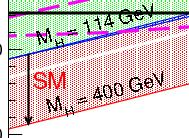So far we have described measurements. Then, there are two different physical models one should consider in order to link those measurements to the Higgs mass. The first one is the Standard Model: it dictates precisely the inter-dependence of all the parameters mentioned above. Because of the precise SM predictions, for any choice of the Higgs boson mass one can draw a curve in the top mass versus W mass plane. However, in the graph a full band is hatched instead. This correspond to allowing the Higgs boson mass to vary from a minimum of 114 GeV to 400 GeV. 114 GeV is the lower limit on the Higgs boson mass found by the LEP II experiments in their direct searches, using electron-positron collisions; while 400 GeV is just a reference value.

The boundaries of the red region show the functional dependence of Higgs mass on top and W masses: an increase of top mass, for fixed W mass, results in an increase of the Higgs mass, as is clear by starting from the 114 GeV upper boundary of the red region, since one then would move into the region, to higher Higgs masses. On the contrary, for a fixed top mass, an increase in W boson mass results in a decrease of the Higgs mass predicted by the Standard Model. Also note that the red region includes a narrow band which has been left white: it is the region corresponding to Higgs masses varying between 160 and 170 GeV, the masses that direct searches at the Tevatron have excluded at 95% confidence level.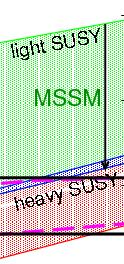The second area, hatched in green, is not showing a single model predictions, but rather a range of values allowed by varying arbitrarily many of the parameters describing the supersymmetric extension of the SM called “MSSM”, its “minimal” extension. Even in the minimal extension there are about a hundred additional parameters introduced in the theory, and the values of a few of those modify the interconnection between top mass and W mass in a way that makes direct functional dependencies in the graph impossible to draw. Still, the hatched green region shows a “possible range of values” of the top quark and W boson masses. The arrow pointing down only describes what is expected for W and top masses if the mass of supersymmetric particles is increased from values barely above present exclusion limits to very high values.

So, to summarize, what to get from the plot ? I think the graph describes many things in one single package, and it is not easy to get the right message from it alone. Here is a short commentary, in bits.

• All experimental results are consistent with each other (but here, I should add, a result from NuTeV which finds indirectly the W mass from the measured ratio of neutral current and charged current neutrino interactions is not shown);
• Results point to a small patch of the plane, consistent with a light Higgs boson if the Standard Model holds
• The lower part of the MSSM allowed region is favored, pointing to heavy supersymmetric particles if that theory holds
• Among experimental determinations, the most constraining are those of the top mass; but once the top mass is known to within a few GeV, it is the W mass the one which tells us more about the unknown mass of the Higgs boson
• One point to note when comparing measurements from LEP II and the Tevatron experiments: when one draws a 2-D ellipse of 68% contour, this compares unfavourably to a band, which encompasses the same probability in a 1-D distribution. This is clear if one compares the actual measurements: CDF$80.413 \pm 48 MeV$ (with 200/pb of data), DZERO$80,401 \pm 44 MeV$ (with five times more statistics), LEP II$80.376 \pm 33 MeV$ (average of four experiments). The ellipses look like they are half as precise as the black band, while they are actually only 30-40% worse. If the above is obscure to you, a simple graphical explanation is provided here.
• When averaged, CDF and DZERO will actually beat the LEP II precision measurement -and they are sitting on 25 times more data (CDF) or 5 times more (DZERO).

## Guest post: Ben Allanach, “Predictions for SUSY Particle Masses”September 4, 2008

Posted by dorigo in cosmology, news, physics, science.
Tags: , ,Ben Allanach is a reader in theoretical physics at the University of Cambridge. Before that he was a post-doc at LAPP (Annecy, France), CERN (Geneva, Switzerland), Cambridge (UK) and the Rutherford Appleton Laboratory (UK). He likes drawing and playing guitar in dodgy rock bands. He is currently interested in beyond the standard model collider phenomenology, and is the author of SOFTSUSY, a computer program that calculates the SUSY particle spectrum. He also tries to do a bit of outreach from time to time. I invited him to discuss the results of his studies here after I discussed the paper by Buchmuller et al. two days ago, since I was interested in understanding the subtle differences between today’s different SUSY forecasts.

In a paper last year “Natural Priors, CMSSM Fits and LHC Weather Forecasts “, we (Kyle Cranmer, Chris Lester, Arne Weber and myself) performed a global fit to a simple supersymmetric model (the CMSSM). Data included were:

• relic density of dark matter
• Top mass, strong coupling constant, bottom mass and fine structure
constant data
• Electroweak data: W mass and the weak mixing angle
• Anomalous magnetic moment of the muon
• B physics:$B_s \rightarrow \mu\mu$ branching ratio,$b \rightarrow s \gamma$ branching ratio, and$B \rightarrow K^* \gamma$ isospin asymmetry
• All direct search limits, including higgs limits from LEP2

and used to make predictions for supersymmetric particle masses and cross sections. We showed two characterisations of the data: Bayesian (with various prior probability measures) and the more familiar frequentist one, which I’ll discuss here.

We vary all parameters in order to produce a profile likelihood plot of the LHC cross-sections for producing either strongly interacting SUSY particles, weak gaugino SUSY particles or sleptons directly. This is equivalently a plot of $latex e^{-\chi^2)/2}$: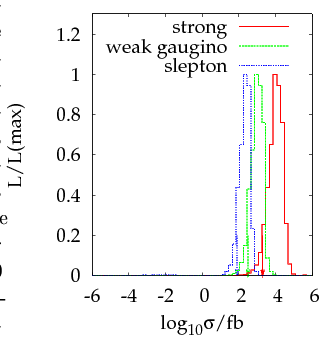The good news is that the LHC has great prospects for producing SUSY particles in large numbers assuming the CMSSM: for 1$fb^{-1}$ of data, we expect the production of over 2000 of them to 95% confidence level (shown by the downward facing arrows). Of these, some fraction will escape detection, but the message is very positive. The CMSSM prefers a light higgs, as shown by this plot:The different curves correspond to different assumptions about the priors (the green one labelled profile shows the usual$\chi^2$ interpretation), but as the figure shows, these aren’t so important. Arrows show the 95% confidence level upper bounds: 118 GeV for the lightest neutral higgs$h$.

### Comparison of results from two papers

The results are quite similar to the recent ones of the Buchmueller et al crowd (who use recent updated data and more observables) lightish SUSY is preferred, primarily because the anomalous magnetic moment of the muon prefers a non-zero SUSY contribution. Also, the W boson mass and weak mixing angle show a slight preference for light SUSY. Because the LHC has enough energy to produce these particles, detection should be quite easy.

The central results of each paper can be expressed in the parameter plane$m_0$ vs$M_{1/2}$ (scalar supersymmetric particle masses vs gaugino supersymmetric particle mass). Here, I show the result of our fit on the left and theirs on the right: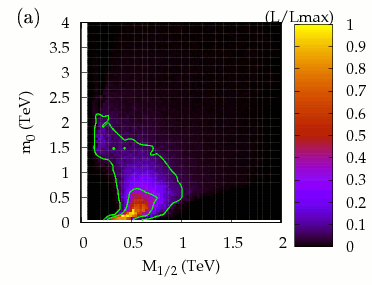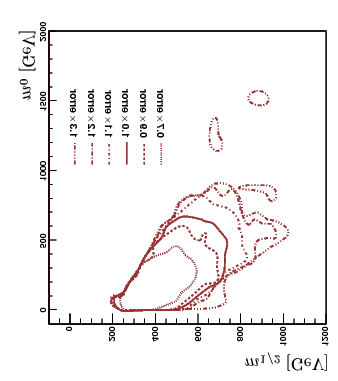To compare the two figures, you must convert their axes of the right-hand figure to the one on the left (note the different scales, although I tried to re-size them to make the scales comparable – apologies to Buchmueller et al for flipping their axes to aid comparison). The comparison should be between the solid line of the right-hand diagram, and the outer solid line on the left (both 95% confidence level contours), but the Buchmueller et al gang get lighter scalars than us, by a factor
of about 2 or so.

### Why should the two results differ?

The top mass has changed in the last year from$m_t=170.9 \pm 1.8$ GeV to$m_t=172.4 \pm 1.2$ GeV. Also, Buchmueller et al include additional observables: other electroweak, B and K-physics ones. My understanding is that none of these is very sensitive to the SUSY particle masses, given the constraints from direct searches though. Perhaps most of these extra observables very slightly prefer light SUSY, so that they disfavour$m_0=1000-2000$ GeV range? Buchmueller et al should be able to tell us by examining their data.

Thanks to Tommaso for inviting this guest post.

## Events with photons, b-jets, and missing EtJune 17, 2008

Posted by dorigo in news, physics, science.
Tags: , , , ,

A recent analysis by CDF, based on 2 inverse femtobarns of data (approximately 160 trillion proton-antiproton collisions) has searched for events featuring a rare mixture of striking objects: high-energy photons, significant missing transverse energy, and energetic b-quark jets. Photons at a proton-antiproton collider are by themselves a sensitive probe of several new physics processes, and the same can be said of significant missing energy. The latter, in fact, is the single most important signature of supersymmetric decays, since the latter usually feature a non-interacting, neutral particle, as I had a chance of explaining in a lot of detail in two posts on the searches for dark matter at colliders (see here for part 1, here for part 2, and here for part 3). Add b-quark jets to boot, and you are looking at a very rare signature within the standard model, but one that may in fact be due to hypothetical exotic processes.

The idea of such a signature-based search is simple: verify whether the sum of standard model processes account for the events observed, without having to be led by any specific model for new physics. The results are much easier to interpret in terms of models that theorists might not have cooked up yet. A specific process which could provide the three sought objects together is not hard to find, in any case: in supersymmetric models where a photino decays radiatively emitting a photon and turning into a Higgsino -a lightest particle which escapes the detector, one gets both photons and missing energy; the additional b-jet is then the result of the decay of an accompanying chargino.

If the above paragraph makes no sense to you, worry not. Just accept that there are possible models of new physics where such a trio of objects arise rather naturally in the final state.

However, there is another, much more intriguing, motivation for the search described below. So let me open a parenthesis.

In Run I, CDF observed a single striking, exceedingly rare event which contained two high-energy electrons, two high-energy photons, and significant missing transverse energy. A unexplicable event by all means! Below you can see a cut-away view of the calorimeter energy deposits: pink bars show electromagnetic energy (both electrons and photons leave their energy in the electromagnetic portion of the calorimeter), but photon candidates have no charged track pointing at them. The event possesses almost nothing else, except for the large transverse energy imbalance, as labeled.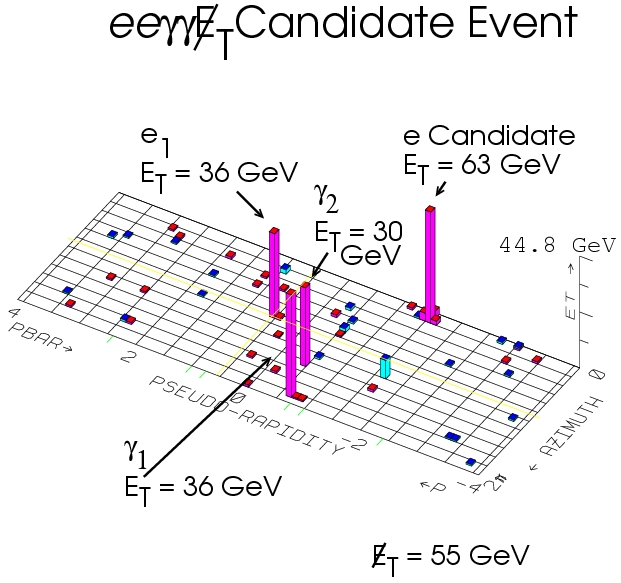The single event shown above was studied with unprecedented detail, and some doubts were cast on the nature of one of the two electron signals. Despite that, the event remained basically unexplained: known sources were conservatively estimated at a total of$1 \pm 1$ millionth of an event! A definitive answer on it was thought would be given by the larger dataset that the Tevatron Run II would soon provide. You can read a very thorough discussion of the characteristics of the infamous$ee \gamma \gamma \not E_t$ event in a paper on diphoton events published in 1999 by CDF.

Closing the parenthesis, we can only say that events with photons and missing transverse energy are hot! So, CDF looked at them with care, by defining each object with simple cuts -such that theorists can understand them. No kidding: if an analysis makes complicated selections, a comparison with theoretical models coming after the fact becomes hard to achieve.

The cuts are indeed straightforward. A photon has to be identified with transverse energy above 25 GeV in the central calorimeter. Two jets are also required, with$E_T>15 GeV$ and$|\eta|<2.0$; Rapidity$\eta$ is just a mesure of how forward the jet is going; a rapidity of 2.0 corresponds to about 30 degrees away from the beam line, if I remember correctly. Selecting these events leads to about 2 million events! These are dominated by strong interactions where a photon is faked by a hadronic jet.

The standard selection is tightened by requiring the presence of missing transverse energy above 25 GeV. Missing transverse energy is measured as the imbalance in the energy flowing in the plane transverse to the beam axis; 25 GeV are usually already a significant amount, which is hard to fake by jets whose energy has been under- or overestimated. The two jets are also required to be well separated between each other and from the photon, and this leads to 35,463 events: missing Et has killed alone about 98% of our original dataset. But missing Et is most of the times due to a jet fluctuation, even above 25 GeV: thus it is further required that it is not pointing along the direction of a jet in the azimuthal angle (the one describing the direction in the plane orthogonal to the beam, which for missing transverse energy is indeed defined). A cut$\Delta \Phi >0.3$ halves the sample, which now contains 18,128 events.

Finally, a b-tagging algorithm is used to search for the secondary vertex B mesons produce inside the jet cones. Only 617 events survive the requirement that at least a jet is b-tagged. These events constitute our “gold mine” and they are interpreted as a sum of standard model processes, to the best of our knowledge.

One last detail is needed: not all the b-tagged jets are originated from real b-quarks! A sizable part of them is due to charm quarks and even lighter ones. To control the fraction of real b-quarks in the sample, one can study the invariant mass of the system of charged tracks which are fit together to a secondary vertex inside the jet axis. The invariant mass of the tracks is larger for b-jets, because b-quarks weigh much more than lighter ones, and their decay products reflect that difference. Below, you can see the “vertex mass” for b-tagged jets in a loose control sample of data (containing photons and jets with few further cuts): the fraction of b-jets is shown by the red histogram, while the blue and green ones are the charm and light-quark components. Please also note the very characteristic “step at about 2 GeV, which is due to the maximum mass of charmed hadrons.The vertex mass fit in the 617 selected events allows to extract the fractions of events due to real photons accompanied by b-jets, c-jets, and fake b-tags (light quark jets). In addition, one must account for fake photon events. Overall, the background prediction is extracted by a combination of methods, well-tested by years of practice in CDF. The total prediction is of$637 \pm 54 \pm 128$ events (the uncertainties are statistical and systematic, respectively), in excellent agreement with observed counts. A study of the kinematics of the events, compared with the sum of predicted backgrounds, provides a clear indication that Standard Model processes account very well for their characteristics. No SUSY appears to be lurking!

Below you can see the missing transverse energy distribution for the data (black points) and a stack of backgrounds (with pink shading for the error bars on background prediction).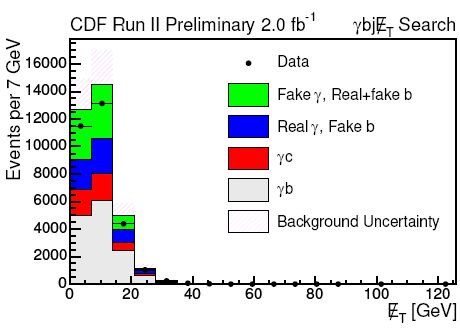Below, a similar distribution for the invariant mass of the two jets.A number of kinematic distirbutions such as those shown above is available in the paper describing the preliminary results. Interested readers can also check the public web site of the analysis.

## An update on the 2.1-sigma MSSM Higgs signalMay 29, 2008

Posted by dorigo in news, physics, science.
Tags: , ,

By doing some cleanup, I discovered this afternoon a forgotten text I had written seven months ago and put in stand-by, awaiting the proper time to post it. The reason for the delay -which is an order of magnitude longer than I had originally intended- is explained in the text highlighted in purple, while in green is any correction and amendment I made to the original post. Anyway, despite the fact that the topic of this post is no longer “new”, I think it remains interesting – and the result described is still the best so far on this channel. So please find the recovered text below. Before it, I chose to re-post the introductory explanation of the physics.

Last January  readers of this blog and Cosmic Variance got acquainted with a funny effect seen by CDF in the data where they were searching for a signal of supersymmetric Higgs boson decays to tau lepton pairs: the data did allow for a small signal of$H \to \tau \tau$ decays, if a higgs mass of about 150-160 GeV was hypothesized, and for a hiterto not excluded value of some critical parameters describing the model considered in the search. The plot below shows the mass distribution of events compatible with the searched double tau-lepton final state: backgrounds from QCD, electroweak, and Drell-Yan processes are in grey, magenta and blue, respectively, and the tentative signal is shown in yellow.Despite John Conway (the writer in CV and one of the analysis authors) and I were quite adamant in explaining that the effect was most likely due to a fluctuation of the data, and that its significance was in all cases very scarce, the rumor of a possible discovery spread around the web, and was eventually picked up in articles which appeared in March on New Scientist and the Economist. I have described in detail the whole process and its implications time and again (check my Higgs search page), so I will not add anything about that here.

What I wish I could discuss today is the new result obtained by John and his team in the same search, which is now based on twice as much statistics. You would guess that if you double the statistics, a true signal would roughly double in size, and its significance would grow by about 40%: Correct. Further, if you also had some experience with hadron collider results, you would actually expect an even larger increase, because analyses in that environment continue to improve as time goes by and a better understanding of backgrounds is achieved. On the other hand, a fluctuation would be likely to get washed away by a doubling of the data…

CDF has a policy of making public a physics result only after a careful internal scrutiny and several passes of review. After the result is “blessed”, there is nothing wrong in distributing it – but a nagging moral responsibility remains toward the very authors, which have to be left the chance of being the first to present their findings to the outside world. I used to not consider this to be a real obligation in the past, until I discussed the matter with a few colleagues. Among them, the same John Conway who is the mastermind behind the$H \to \tau \tau$ analysis. I have a high esteem of John, which I maturated during a decade of collaboration; he was instrumental in making me change my mind about the issue. For that reason, I am not able to disclose the details of his brand new result here, which was blessed last week in CDF, until I get news about a public talk on the matter.

Because of the above, this post will not discuss the details of the new result, and it will remain unfinished business for a while. I will update it with the description of the result when I have a green light; for the time being, I think I can still do something useful though: make an attempt at putting readers in the condition of understanding the main nuts and bolts of the theoretical model within which the 2.1 sigma excess was found nine months ago.

I will describe the new result below, but first let me introduce the topic and put it in context.

1 – TWO WORDS ABOUT SUSY

First of all, what is the MSSM ? MSSM stands for “Minimal SuperSymmetric Model”. It is an extension of the Standard Model of particle physics which attempts a solution of some of its wanting features; it is the minimal version of a class of theories called SUperSYmmetric – SUSY for friends. These theories postulate a symmetry between fermions (particles having a half-integer value of the quantum number called “spin”) and bosons (particles with zero or integer spin): for every known fermion (spin 1/2) there exists a supersymmetric partner, whose characteristics are the same except for having spin 1; and likewise for bosons (spin 1). Such a doubling of all known particles would allow to automatically solve the problem of “fine tuning” of the Standard Model (which was excellently explained by Michelangelo Mangano recently; also see Andrea Romanino’s perspective on the issue), and it would have the added benefit of allowing a unification of coupling constants for the different interactions at a common, yet very high energy scale. Some say SUSY would make the whole theory of elementary particles considerably prettier; others disagree. If you ask me where I stand, I think it just makes things messier.

Physicists have always been wary of adding parameters or entities to their model of nature, even the model is obviously incomplete or when the addition appears justified by experimental observation. Scientific investigation proceeds well by following Occam’s principle: “entia non sunt multiplicanda praeter necessitatem“, entities should not be multiplied needlessly.

The extension of the standard model to SUSY not only implies the existence of not just one but a score of new, as-of-yet unseen elementary particles: in order for SUSY to be there and still yet to be discovered, we need to have so far missed all these bodies, and the only way that is possible is if all SUSY particles have large masses – so large that we have so far been unable to produce them in our accelerators. Such a striking difference between particles and s-particles can be due to a “SUSY-breaking” mechanism, a contraption by which the symmetry between particles and sparticles is broken, endowing all sparticles with masses much larger than that of the corresponding particles: and funnily enough, their value has to be juuuuust right above the lower limits set by direct investigation at the Tevatron and elsewhere, in order for the coveted “unification of coupling constants” to be possible.

So if we marry the hypothesis of SUSY, we need to swallow the existence of a whole set of new bodies AND a uncalled-for mechanism which hid them from view until today. Plus, of course, scores of new parameters: mass values, mixing matrix elements, what-not. Occam’s razor is drooling to come into action. In fact, so many choices are possible for the free parameters of the theory, that in order to be sure of talking about the same model phenomenologists have conceived some “benchmark scenarios”: choices of parameters that describe “typical” points in the multi-dimensional parameter space.

2 – THE HIGGS SECTOR OF THE MSSM

A very important subclass of these benchmarks (but some would frown at my calling a benchmark: it is more like a space of models) is the so-called “Minimal Supersymmetric extension” of the standard model, also known as MSSM. In the MSSM the Higgs mechanism yields the smallest number of Higgs bosons: five physical particles, as opposed to a single neutral scalar particle in ths standard model. Let me introduce them:

• two neutral, CP-even states: h, H (with$m_h < m_H$)
• one neutral, CP-odd state, A
• two electrically charged CP-odd states:$H^+, H^-$.

The CP-parity of the states need not bother you. It is irrelevant for the searches discussed in this post. However, you should take away the fact that there are three, and not just one, neutral scalar boson to search for.

Where do these five states come from ? Well, the symmetry structure of SUSY requires that two different higgs boson doublets are responsible for the mass of up-type (u,c,t quarks and e,$\mu$, and $\tau$ leptons) and down-type (d,s,b quarks and the three neutrinos) fermions. Two (2) doublets (x2) of complex (x2) scalar fields make for a total of eight degrees of freedom – eight different real numbers, to be clear; three of these are spent to give rise to masses of W and Z bosons by the higgs mechanism, and five physical particles remain.

There are a few interesting “benchmarks” in the MSSM. One is called no mixing scenario, and is the one most frequently used by experimentalists – mainly because it is one of the most accessible by direct searches. There are quite a few others: “Mh max”, “Gluophobic Higgs”, “Small$\alpha(eff)$… but we need not discuss them here. What matters is that once the no mixing scenario or any other has been selected, just two additional parameters are necessary to calculate the masses and couplings of the five higgs bosons: the mass of the A boson,$m_A$, and$tan(\beta)$, a ratio between the characteristics of the two higgs doublets.

It turns out that if$tan (\beta)$ is large, then the production rate of higgs bosons can be hundreds of times higher than that predicted in the standard model! Of course, very large values of$tan(\beta)$ have already been excluded by direct searches because of that very feature: if no higgs bosons have been found this far, then their production rate must be smaller than a certain value, and that translates in an upper bound for$tan(\beta)$. Nonetheless the parameter space – usually plotted as the plane where the abscissa is$m_A$ and the y-axis represents$tan(\beta)$ – is still mostly to be explored experimentally. Below you can see the excluded region by the analysis of Conway et al. in January 2007.One thing to keep in mind when discussing the phenomenology of these theories is the following: among the three neutral scalars, a pair of them ([h,A] or [H,A]) are usually very close in mass, such that they effectively add together their signals, which are by all means indistinguishable. Therefore, rather than discussing the search for a specific state among h, H, and A, experimentalists prefer to discuss a generic scalar$\phi$, a placeholder for the two degenerate states.

3 – MSSM HIGGS PRODUCTION AND DECAY

Higgs production in the MSSM is not too special: the diagrams producing a neutral scalar (h, H, or A) are the same. However, due to the highly boosted couplings of two of these three states with down-type fermions (an increase roughly equal to$tan(\beta)$, two diagrams contribute the most: gluon-gluon fusion via a b-quark loop (see below, left) or direct b-quark annihilation (right). The b-quark in fact is privileged by being a down-type quark AND having a large mass.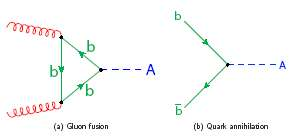As for the decay of these particles, the same enhancement in the couplings rules that the most likely decay is to b-quark pairs (about 85 to 90%). The remainder is essentially a 10-15% chance of decay to tau-lepton pairs, which are also down-type fermions and also have a largish mass: 1.777 GeV, to be compared to the about 3-4 GeV of b-quarks “photographed” at high$Q^2$. Decay rates scale with the square of the coupling, and the coupling scales with the mass: that explains the order of magnitude difference in decay rates.

Because of the impossibility of going on to describe the analysis, I will conclude this incomplete post with a point about the parameter space. [Let’s see this anyway before I describe the result]. There is in fact one subtlety to mention. As$tan(\beta)$ becomes large, the usually narrow higgs bosons acquire a very large width. The width of a particle is an attribute which defines how close to the nominal mass the actual mass of the state can be. Now, the higgs boson in the standard model has a width much smaller than 1 GeV, which is totally irrelevant when compared with the experimental precision of the mass reconstruction. The same cannot be said for MSSM higgs bosons if$tan(\beta)$ is large: it is the large coupling to down-type fermions the cause of the large indetermination in the mass, in fact. As$tan(\beta)$ grows larger than about 60, the coupling actually becomes non-calculable by perturbation theory, the width becomes really large and rather undetermined (10 GeV and above), and the higgs resonances lose their most significant attribute, i.e. a well-defined mass.

The effect discussed above has two consequences: one is that the region of parameter space corresponding to too large values of$tan(\beta)$ is not well-defined theoretically. The other is that if one were to perform the search carefully in that region, one would need to consider the effect of the large width to the mass templates used to search for the higgs bosons. Given a mass of the A particle, a different mass template would be then needed for each value of$tan(\beta)$, making the analysis quite a bit more complex. Physicists like to approximate, and mostly they get away with it when the neglected effects are small, but in the case of large$tan(\beta)$ an approximation fails and a precise computation is not possible.

The bottomline is: a grain of salt is really needed when interpreting the results of a MSSM Higgs search.

That said, I think it is time for a rapid description of the analysis.

4 – THE EXPERIMENTAL SEARCH BY CDF

CDF and D0 have been searching for MSSM neutral higgs bosons for a while now. I reported about the latest result by CDF, obtained from the analysis of events with three b-quark jets, [just a month ago] last fall. Now it is the time for the brand new CDF search for the decay$H \to \tau \tau$, which in January 2007 made headlines due to the excess it was showing.

The analysis was not modified appreciably from its former instantiation. However, I took the time to read the internal analysis note describing in detail the studies which the authors performed in order to understand the data and tune the selection cuts, and I must say I was really impressed by the amount and quality of the work they performed. I have to tip my hat to John Conway – the person who has been after this signature of higgs decay since more than a decade ago – and to Anton Anastassov, also a tau identification expert and a renowned Higgs hunter. Other authors are Cristobal Cuenca, Dongwook Jang, and Amit Lath.

I was mentioning that the analysis has stayed the same during 2007: indeed, it was a very good idea, in order to avoid a potential signal from being washed away by a modified analysis – although I feel urged to say that a genuine signal cannot hide forever and is bound to creep out of the data at some point!

A total of 1.8 inverse femtobarns of data were analyzed. These correspond to a hundred thousand billion collisions, as I have grown tired of mentioning: among these, a online trigger system selected those containing a likely signal of an electron or muon. Tau leptons do in fact decay to these lighter leptons about a third of the times:$\tau \to e \nu_e \nu_\tau$,$\tau \to \mu \nu_\mu \nu_\tau$. Yes: you read it correctly: they yield an electron or a muon accompanied by two neutrinos. The latter provide no chance of detection.

And what about the remaining two thirds ? These are “hadronic” decays: tau leptons yield a narrow jet of light hadrons in the remaining cases. These jets contain few charged particles (typically one or three) and leave a signal in the calorimeter which refined algorithms can distinguish – but only on a statistical basis – from jets originated by fragmenting quarks and gluons.

If one is looking for two tau leptons from the decay of a$\phi$ neutral scalar boson, one has to decide which kind of decay of the taus to look for. Electrons and muons provide a clean signature but are rather infrequent, while hadrons are more frequent but also background-ridden. The analysis considers three final states that are a good compromise of rate and background contamination:

1.$\tau_e \tau_\mu$
2.$\tau_e \tau_h$
3.$\tau_\mu \tau_h$

where subscripts indicate the decay to electrons, muons, or hadrons. Double decay to electrons and double decay to muons are neglected because of the large background from Drell-Yan production of lepton pairs, and double decay to hadrons is not considered due to the too large background.

One thing that should be clear is that all three final states contain at least two tau neutrinos:$\nu_\tau$ are in fact the final product of the decay chain in all cases; the semi-leptonic final states 2. and 3. also contain an additional$\nu_e$ or$\nu_\mu$, respectively; and the dileptonic final state 1. yields a total of four neutrinos. Neutrinos make the event reconstruction tough, because they escape carrying away energy and momentum, and making a direct reconstruction of the invariant mass of the body producing the two tau leptons impossible.

Despite that problem, a partial reconstruction of the mass of the tentative Higgs boson producing the taus is possible by using only the visible energy – that of electrons, muons, and jets, plus the so-called “missing transverse energy”, a vector equal to the imbalance in transverse momentum obtained by vectorially adding together all visible transverse momenta. The reason for only considering the transverse component is due to the fact that in hadron collisions the longitudinal motion of the initial state – i.e., the speed of the center of mass of the collision along the beam direction – is not known: it is not a proton and an antiproton of 980 GeV each that collide, but rather a quark and a antiquark, each of unknown energy.

The figure below shows that a discrimination between Higgs boson signals of different masses is indeed possible: a$Z \to \tau \tau$ decay yields a signal which, once reconstructed, looks like the empty histogram, while higgs bosons of 115 and 200 GeV give the distributions pictured in blue and magenta, respectively.

A selection of tau candidates in the three detection categories is performed by requiring electrons and muons to be well-identified and isolated, while jets have to be narrow, contain only one or three charged tracks, and be flagged by a fine-tuned tau-identification algorithm. Then, the kinematics of the event is also required to be Higgs-like, by exploiting angular dependence of missing transverse energy and tau decay candidates.

After the selection, the invariant mass distribution of candidates in the three search categories are finally understood as a sum of the different background processes contributing to the selected data. A refined likelihood fitting technique incorporating systematic uncertainties as nuisance parameters and template morphing method to account for jet energy scale uncertainty provides a quite accurate determination of the amount if signal allowed by the data, as a function of the mass of the CP-odd state$m_A$. Below are shown the fits in the category$\tau_X \tau_h$ (top, where X =$e, \mu$) and$\tau_e \tau_\mu$ (bottom). The abscissa is the reconstructed visible mass of the tau pair, the black points are experimental data, and the various histograms stacked one on top of the other show the expected amount of QCD background from fake tau signals (red), electroweak and top pair backgrounds (blue), Drell-Yan dilepton production including Z decays (white), and in yellow the amount of$\phi$ signal that the data excludes at 95% confidence level.As is clear by looking at the plots, the data follow quite well the sum of backgrounds in both datasets, and no signal is apparent. And in fact, the cross section limit (red curve in the graph below) follows very closely the expectation calculated from pseudoexperiments (hatched curve, with 1- and 2-sigma blue and grey bands overlaid):From the cross section limit, an exclusion limit in the plane of A mass and tan(beta) can be determined. This is much more stringent than the one John and company obtained in January 2007, and it provides a significant advance in our investigation of supersymmetric models.So, a question remains to be answered at the end of this longish post. And that is, How should we interpret the$2.1 \sigma$ excess that Conway et al. found in their former analysis ? Of course, as a statistical fluctuation! One that happens roughly once in a hundred cases. Discovering a supersymmetric higgs, on the other hand, is something that can only happen once in a lifetime. Not in this lifetime, if you ask me.

## Dinner with Gordie KaneMay 23, 2008

Posted by dorigo in personal, physics, science.
Tags: , , ,